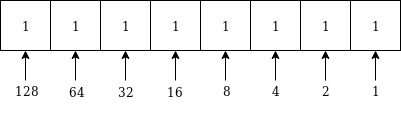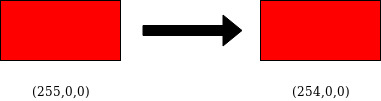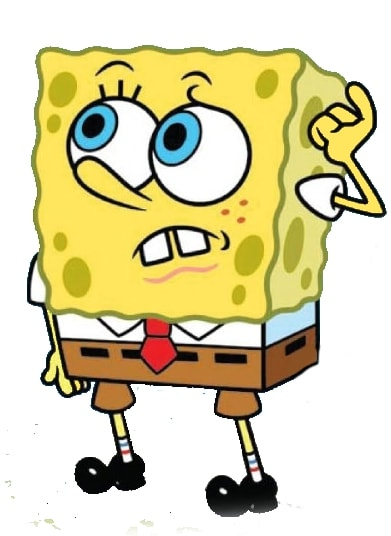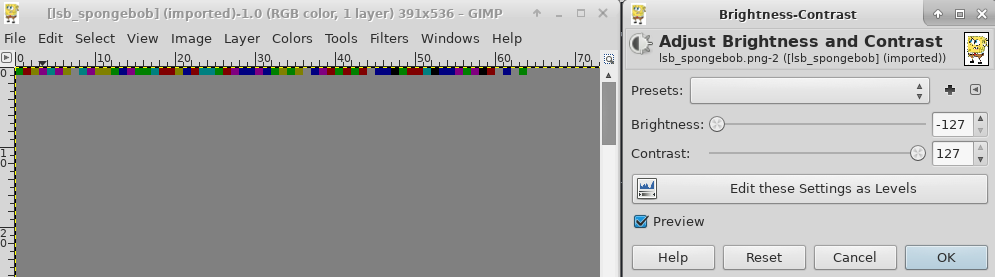This article details a common steganography method known as the Least Significant Bit. This technique is very efficient because of its simplicity and its ability to be undetectable to the naked eye. After reading this, you’ll be able to hide a message inside a picture using this technique, but also to detect any dissimulated message.

## Do It Yourself!

If you’re already familiar with the concept of LSB and simply want to practice, download this picture and feel free to send me your result or to post it in the comment section.

## Technical description

### Digital image structure

To understand this technique, a few reminders of some digital imaging basics might be useful.

• A digital image is composed of $X$ rows by $Y$ columns.
• The point of coordinates $[a,b]$ with $0\leqslant a<X$ and $0\leqslant b<Y$, is called a pixel. The pixel represents the smallest addressable element of a picture.
• Each pixel is associated with a color, usually decomposed in three primary colors: Red, Green, Blue. A pixel can then be specified as pixel(Red, Green, Blue), that’s what we call the RGB model.
• Red, Green and Blue intensities can vary from 0 to 255.
• WHITE = (255,255,255) and BLACK = (0,0,0).
• A pixel take 3 bytes of memory, 1 for each primary component (hence the maximum value of 255).
• A byte consists of 8 bits, representing a binary number (example: 1010 0101).
• The highest value a byte can take is 1111 1111, which is equal to 255 in decimal.

### LSB Principle

Now that you have the structure of a digital image in mind, we can start talking about the serious stuff.

As its name suggests, the Least Significant Bit technique is based on hiding information in the least significant bit of each byte of the picture. There are multiple variants of LSB but, in this article, we will set the focus on the most common one.

#### Am I not significant to you?

The notion of “Least Significant Bit” probably doesn’t speak to everyone so I’ll explain it. Let’s take the following representation of a byte, where the weight is annotated below each bit:The first bit on the left is the “heaviest” one since it’s the one that has the biggest influence on the value of the byte. Its weight is 128.

Now look at the bit on the very right. Its weight is 1 and it has a very minor impact on the value of the byte. In a way, this bit is the least significant bit of this byte.

#### Why do we modify this very specific bit?

Well, simply because it’s the least significant one. Let me explain:

The following diagram illustrates the color difference when the least significant bit of the red channel is modified.Can you spot the difference? No? Me neither and that’s exactly the goal! That way, we can modify 3 bits per pixel without it is noticeable.

### How to hide a message?

Ok the theory should be clear now, but we’ve seen that we can only hide 3 bits per pixel and we want to dissimulate a full message! How are we supposed to do?

Easy! A message is actually a sequence of bits so it’s not an issue. The only limitation is that the size of the message in bits must be inferior to the number of pixels in the picture multiplied by 3.

There are plenty of tools already available for hiding a message inside a picture with the LSB technique but I encourage you to write your own tool. This will help you getting familiar with a scripting language and will require from you a prefect understanding of the concept.

For the needs, of this tutorial I used Python 2.7. Sources of the scripts used in this article will be downloadable at the bottom of the page.

Alright, let’s dive into the code!#### Encoding and transforming a string into a sequence of bits

In order to avoid data losses caused by encoding problems, the initial message must be base64-encoded. There are many ways to turn a string into its binary representation in python, but I decided to use the bitarray module. If you don’t have it installed, just type sudo pip install bitarray.

import bitarray
import base64

message = 'YourVerySecretText'
encoded_message = base64.b64encode(message)
#Converts the message into an array of bits
ba = bitarray.bitarray()
ba.frombytes(encoded_message.encode('utf-8'))
bit_array = [int(i) for i in ba]


bit_array now contains the binary representation of our message.

NOTE: Make sure to hide your message inside a PNG file and not a JPEG or its lossy compression algorithm will overwrite your modifications!

#### Messing with pixels

Let’s say we want to hide our message inside this picture (download with Right click > Save Image as…):There’s a wonderful python library for manipulating images called PIL (pillow since python3).

First, let’s duplicate the original picture. We will only modify the one called “lsb_spongebob.png”. Then, we store the image size for later. The load() function retrieves an array containing every pixel in RGB format.

from PIL import Image

im = Image.open("spongebob.png")
im.save("lsb_spongebob.png")

im = Image.open("lsb_spongebob.png")
width, height = im.size


Let’s say we want to hide our message at the beginning of the first row of the picture, I’ve written the following piece of code which is kinda ulgy, I agree, but that makes the job, you know.

i = 0
for x in range(0,width):
r,g,b = pixels[x,0]
print("[+] Pixel : [%d,%d]"%(x,0))
print("[+] \tBefore : (%d,%d,%d)"%(r,g,b))
#Default values in case no bit has to be modified
new_bit_red_pixel = 255
new_bit_green_pixel = 255
new_bit_blue_pixel = 255

if i<len(bit_array):
#Red pixel
r_bit = bin(r)
r_last_bit = int(r_bit[-1])
r_new_last_bit = r_last_bit & bit_array[i]
new_bit_red_pixel = int(r_bit[:-1]+str(r_new_last_bit),2)
i += 1

if i<len(bit_array):
#Green pixel
g_bit = bin(g)
g_last_bit = int(g_bit[-1])
g_new_last_bit = g_last_bit & bit_array[i]
new_bit_green_pixel = int(g_bit[:-1]+str(g_new_last_bit),2)
i += 1

if i<len(bit_array):
#Blue pixel
b_bit = bin(b)
b_last_bit = int(b_bit[-1])
b_new_last_bit = b_last_bit & bit_array[i]
new_bit_blue_pixel = int(b_bit[:-1]+str(b_new_last_bit),2)
i += 1

pixels[x,0] = (new_bit_red_pixel,new_bit_green_pixel,new_bit_blue_pixel)
print("[+] \tAfter: (%d,%d,%d)"%(new_bit_red_pixel,new_bit_green_pixel,new_bit_blue_pixel))

im.save('lsb_spongebob.png')


What this script does is actually pretty simple. For each color channel of each pixel of the first row, the script extracts the least significant bit and replaces it by the result of the logical operation & between the current least significant bit and the bit stored at index [i] in bit_array. Once the message is fully written, remaining pixels on the row are replaced by white pixels(255,255,255).

I’ve also added some debugging outputs which are useful in order to illustrate the changes that are being made.

This script only works for hiding short messages in the first row of the picture. It’s not optimized at all so you’ll probably write a better one but you get the idea.

## Detection

If everything went well, our message is now hidden inside “lsb_spongebob.png”. We will now study one specific method allowing us to detect such steganography techniques. There are many others which have a more mathematical approach but, since it’s not my speciality, I won’t mention them here.

The technique I’ll present you is very manual. It consists in playing with brightness and contrast parameters in your favorite (GNU) Image Manipulation Program, in order to spot certain irregularities. Nothing better than a concrete example. I personally use GIMP for this purpose.

• Let’s open “lsb_spongebob.png” with GIMP and open the Brightness-Contrast box under Colors menu.
• Set brightness to its minimum value and contrast to its maximum value.
• Zoom in and scan for irregularities.
• On the top left, you should see something like that:That’s really suspicious because every pixel should be white in this area.

This technique is not 100% reliable but pretty straight-forward and simple.

Once we’ve located the suspected hidden message, we can proceed to the extraction.

## Extraction

We’ve detected LSB steganography inside a picture! But how can we recover the message? Simple! We have to extract the LSBs from each pixel and then assemble the result as a string.

Once again, I recommand you to write your own script because it’s the only way to make sure everything is clear in your mind. In case you encounter difficulties, you can always take inspiration from mine.

### Python my love

We know that the secret is hidden in the first row, so it’s useless to iterate over the whole picture with our script.

#coding: utf-8
import base64
from PIL import Image

image = Image.open("lsb_spongebob.png")

extracted = ''

# Iterate over pixels of the first row
for x in range(0,image.width):
r,g,b = pixels[x,0]
# Store LSB of each color channel of each pixel
extracted += bin(r)[-1]
extracted += bin(g)[-1]
extracted += bin(b)[-1]

chars = []
for i in range(len(extracted)/8):
byte = extracted[i*8:(i+1)*8]
chars.append(chr(int(''.join([str(bit) for bit in byte]), 2)))

# Don't forget that the message was base64-encoded
flag = base64.b64decode(''.join(chars))
print flag


To make this tutorial a bit funnier, I’ve slightly modified lsb_spongebob.png and I’ve hidden a different message inside. Will you be able to recover it?Maybe this one isn’t exactly in the same place…BoiteAKlou